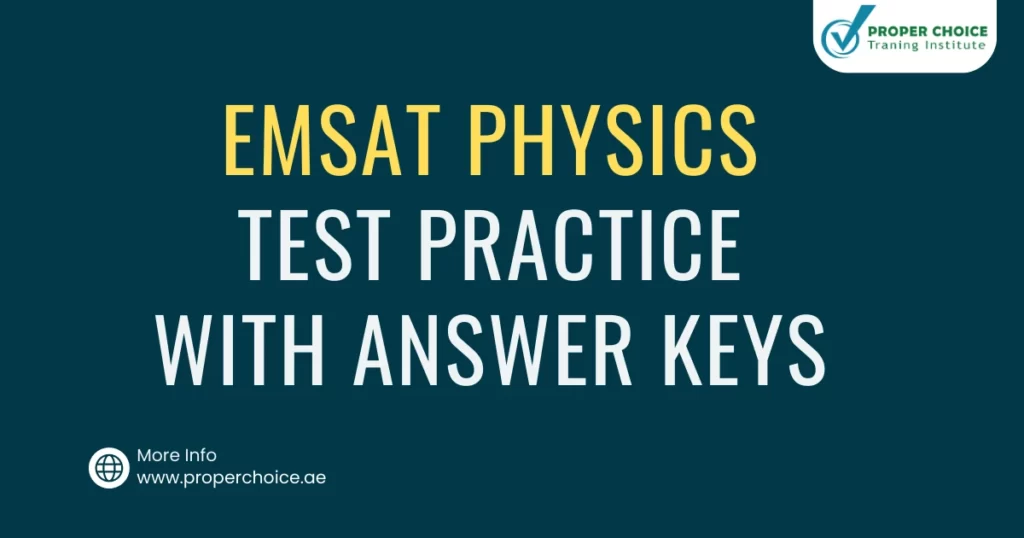# EmSAT Physics test practice with answer keys 2023Last updated on May 14th, 2023 at 09:03 pm

In this blog post, we are providing 10 EmSAT physics practice tests with answers key.

1.  A woman riding bicycle collides head-on with parked school bus. If mass of the woman is one tenth the mass of the school bus. Which object feels greater acceleration.

• A. Woman
• B. School bus
• C. Both feels equal acceleration
• D. None

2. A can of paint with a mass of 10 kg hangs from a rope. If can is to be pulled up to the rooftop with an acceleration of 1.8 m/s2, what must be the tension in the rope? (Use g = 10 m/s2)

• A. 100 N
• B. 118 N
• C. 18 N
• D. 1080 N

3. A crate of mass 100 kg rests on the floor. If the coefficient of static friction is 0.3. If a force of 200 N (parallel to the floor) is applied to the crate. What is the magnitude of the force of static friction on the crate?

• A. 294 N
• B. 250 N
• C. 210 N
• D. 200 N

4. The potential energy stored in an object due to change in tits shape is called

• A. Gravitational Potential Energy
• B. Potential Energy
• C. Elastic Potential Energy
• D. Kinetic Energy

5. The amount of heat needed to melt 1 kg of substance is called

• A. Latent heat of vaporization
• B. Latent heat of fusion
• C. Latent heat
• D. Melting point

6. A gas tank contains 200L of hydrogen 273K and 1M pa. What will be the new pressure if temperature was raised to 373K and volume was reduced to 100L?

• A. 2.73 M Pa
• B. 2.73 K Pa
• C. 0.273 G Pa
• D. 273 G Pa

7. A standing wave produce in a rope that has a frequency of 6 Hz and wave speed of 15 m/s, what is the wavelength?

• A. 1.5 m
• B. 2.5 m
• C. 3 m
• D. 3.5 m

8. If the distance between two-point charges double, the electrostatic force between them will be

• A. Doubles
• B. Increases by two times
• C. does not change
• D. decreases by four times

9. The unit of measuring capacitance is

• A. Volt
• B. Ampere
• D. Coulomb

10. An electric heater with resistance of 10 ohm works on potential difference equal to 120 V. The amount of thermal energy produced in 10 seconds is

• A. 120 J
• B. 1200 J
• C. 14400 J
• D. 24000 J